Next: Selecting atoms for colvars: Up: Defining collective variables and Previous: Extended Lagrangian.   Contents   Index

## Statistical analysis of collective variables

When the global keyword analysis is defined in the configuration file, run-time calculations of statistical properties for individual colvars can be performed. At the moment, several types of time correlation functions, running averages and running standard deviations are available.

• corrFunc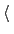Calculate a time correlation function?Context: colvar
Acceptable values: boolean
Default value: off
Description: Whether or not a time correlaction function should be calculated for this colvar.

• corrFuncWithColvarColvar name for the correlation functionContext: colvar
Acceptable values: string
Description: By default, the auto-correlation function (ACF) of this colvar,, is calculated. When this option is specified, the correlation function is calculated instead with another colvar,, which must be of the same type (scalar, vector, or quaternion) as.

• corrFuncTypeType of the correlation functionContext: colvar
Acceptable values: velocity, coordinate or coordinate_p2
Default value: velocity
Description: With coordinate or velocity, the correlation function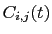=is calculated between the variablesand, or their velocities.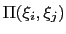is the scalar product when calculated between scalar or vector values, whereas for quaternions it is the cosine between the two corresponding rotation axes. With coordinate_p2, the second order Legendre polynomial,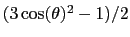, is used instead of the cosine.

• corrFuncNormalizeNormalize the time correlation function?Context: colvar
Acceptable values: boolean
Default value: on
Description: If enabled, the value of the correlation function at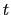= 0 is normalized to 1; otherwise, it equals to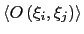.

• corrFuncLengthLength of the time correlation functionContext: colvar
Acceptable values: positive integer
Default value: 1000
Description: Length (in number of points) of the time correlation function.

• corrFuncStrideStride of the time correlation functionContext: colvar
Acceptable values: positive integer
Default value: 1
Description: Number of steps between two values of the time correlation function.

• corrFuncOffsetOffset of the time correlation functionContext: colvar
Acceptable values: positive integer
Default value: 0
Description: The starting time (in number of steps) of the time correlation function (default:= 0). Note: the value at= 0 is always used for the normalization.

• corrFuncOutputFileOutput file for the time correlation functionContext: colvar
Acceptable values: UNIX filename
Default value:name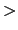.corrfunc.dat
Description: The time correlation function is saved in this file.

• runAveCalculate the running average and standard deviationContext: colvar
Acceptable values: boolean
Default value: off
Description: Whether or not the running average and standard deviation should be calculated for this colvar.

• runAveLengthLength of the running average windowContext: colvar
Acceptable values: positive integer
Default value: 1000
Description: Length (in number of points) of the running average window.

• runAveStrideStride of the running average window valuesContext: colvar
Acceptable values: positive integer
Default value: 1
Description: Number of steps between two values within the running average window.

• runAveOutputFileOutput file for the running average and standard deviationContext: colvar
Acceptable values: UNIX filename
Default value:name.runave.dat
Description: The running average and standard deviation are saved in this file.Next: Selecting atoms for colvars: Up: Defining collective variables and Previous: Extended Lagrangian.   Contents   Index
vmd@ks.uiuc.edu